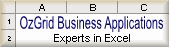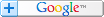Excel Templates - Excel Training - Excel Add-ins

# OzGrid Excel and VBA Newsletter

### Excel Tips and Tricks

For this months Excel tips and tricks we will look at one of the most popular asked questions. That is, how to count using multiple criteria and how to sum using multiple criteria.

*Count/Sum With Multiple Criteria*

They are quite a few ways this can be achieved, but some ways are more efficient than others. Let's Start with the least efficient, but the easiest to do - array formulas.

What are Array Formulas?

Excel Array formulas are very powerful and useful formulas that allow more complex calculations than standard formulas. The "Help" in Excel defines them as below:

"An array formula can perform multiple calculations and then return either a single result or multiple results. Array formulas act on two or more sets of values known as array arguments."

Before we show some examples of array formulas it is important to know four fundamental rules.

1) Each argument within an array must have the same amount of rows and/or columns.

2) You must enter an array by pushing Ctrl+Shift+Enter. This will add the curly brackets around the formula.

3) You cannot add the {} (braces) that surround an array yourself, pushing Ctrl+Shift+Enter will do this for you.

4) You cannot use an array formula on an entire column.

For the purpose of the examples I will base all of them on the data being in columns A:D down to row 100, with row 1 of all columns being a heading. Column A will be Names, B will be Age, C will be Male/Female (M/F), D will be Wage.

Count of; Name=Dave, Age>20 M/F=M, Wage>500
=SUM(((A2:A100="Dave")*(B2:B100>20)*(C2:C100="M")*(D2:D100>500)))

Count of; Age between 21 and 29, Wage between 301 and 399
=SUM((B2:B100>20)*(B2:B100<30)*(D2:D100>300)*(D2:D100<400))

Although these examples use the SUM function the results are counted and not summed. This is because the SUM function sums the results of the multiplication of TRUE (has a value of 1) and FALSE (has a value of 0). So, any row that has a FALSE value will result in the value of zero. As including zero in the multiplication always yields a result of zero, one FALSE means a result of zero. If all are TRUE, then it ends up being SUM(1*1*1) which of course always equals 1.

The other way, which is more efficient, is by using the SUMPRODUCT function. It too uses the same principle as described above for arrays. However, one DOES NOT need to enter the SUMPRODUCT via Ctrl+Shift+Enter

Count of; Name=Dave, Age>20 M/F=M, Wage>500
=SUMPRODUCT((A2:A100="Dave")*(B2:B100>20)*(C2:C100="M")*(D2:D100>500))

Count of; Age between 21 and 29, Wage between 301 and 399
=SUMPRODUCT((B2:B100>20)*(B2:B100<30)*(D2:D100>300)*(D2:D100<400))

Now, to use the same data to SUM values based on multiple criteria, one can use the SUM with the IF function nested within. As we only have meaningful numbers in Column D (Wage), this will be the column we SUM.

Sum of; Name=Dave, Age>20 M/F=M, Wage between 201 and 399
=SUM(IF(A2:A100="Dave",IF(B2:B100>20,IF(C2:C100="M",IF(D2:D100>200,IF(D2:D100<400,D2:D100))))))

=SUMPRODUCT((A2:A100="Dave")*(B2:B100>20)*(C2:C100="M")*(D2:D100>200)*(D2:D100<400)*(D2:D100))

Again, the use of the SUMPRODUCT function is more efficient.

IMPORTANT: BE WARNED, the over-use of either array formulas, or the SUMPRODUCT with multiple criteria WILL result in a dramatic slow down in Excel's calculation and re-calculation. Basically, it comes down to the total number of cells being used in the arrays and/or Sumproducts.

If you are going to be counting, or summing a large number of cells I would advise strongly to use the appropriate Dfunction (Database Function). These are designed specifically for the job of using multiple criteria. The Excel help has lots of information and details on these functions, just search for "Database Functions".

Download Working Examples of DCOUNT, DSUM and DGET used in combination with Excel's Validation feature.

### Excel VBA Tips and Tricks

As we did last month, I'm going to include what appears to be the most popular selections of VBA codes from our site, please enjoy and don't forget to use our free question and answer forum for Excel Help .

This UDF was written by myself to take the place of VLOOKUP when you need to look across ALL the Worksheets in the active Workbook. As with the Standard VLOOKUP, it stops at the first match.

Function VLOOKAllSheets(Look_Value As Variant, Tble_Array As Range, _
Col_num as Integer, Optional Range_look as Boolean)

''''''''''''''''''''''''''''''''''''''''''''''''
'Written by OzGrid.com

'Use VLOOKUP to Look across ALL Worksheets and stops _
at the first match found.
'''''''''''''''''''''''''''''''''''''''''''''''''
Dim wSheet As Worksheet
Dim vFound

On Error Resume Next

For Each wSheet In ActiveWorkbook.Worksheets
With wSheet
vFound = WorksheetFunction.VLookup _
(Look_Value, Tble_Array, _
Col_num, Range_look)
End With
If Not IsEmpty(vFound) Then Exit For
Next wSheet

Set Tble_Array = Nothing
VLOOKAllSheets = vFound
End Function

To use this code, do this:

1. Push Alt+F11 and go to Insert>Module
2. Copy and paste in the code.
3. Push Alt+Q and Save.

Now in any cell put in the Function like this:

=VLOOKAllSheets("Dog",C1:E20,2,FALSE)

Where "Dog" is the value to find.
C1:E20 is the range to look in the first column and find "Dog".
2 is the relative column position in C1:E20 to return return our result from.
FALSE (or ommited) means find and exact match of "Dog".

In other words the UDF has the exact same syntax as Excels VLOOKUP. The only difference is that it will look in ALL Worksheets and stop at the first match. You can find the UDF (VLOOKAllSheets) in the Paste Function dialog (Shift+F3) within the "Function category" of "User Defined".
============================================================================

One very common problem encountered by most Excel users is the correct entering of Excel dates. When a date is not entered in the correct format, Excel will not recognize it as a valid date. This often means you cannot perform calculations with the 'so-called' dates. It also means any charts, Pivot tables etc based off the dates will not be valid. While the use of Excels Validation can help with this it is far from bullet proof. Below is a quite simple way we can have all our dates entered in Excel correctly. Just follow the steps and you will be up and running in 10 minutes. Download Demo , Or Download Advanced Demo ,  where you can add x days, months and years to any date.

1)Open the workbook for the calendar. It is a good idea to use your Personal.xls for this, in which case you should first go to Window>Unhide

2)Go to Tools>Macro>Visual Basic Editor (Alt+F11).

3)Go to Insert>UserForm from within the VBE. This should automatically display the Control Toolbox, if not go to View>Toolbox

4)Right click on the Toolbox and select Additional Controls

5)Scroll through the list until you see: Calendar Control 10.0 (number will differ depending on Excel version), check the checkbox and click OK.

6)Now click the Calendar that is now part of the Toolbox and then click on the UserForm we inserted in step 3.

7)Use the size handles on both the UserForm and the Calendar Control to make them both a reasonable size.

8)Now ensure the UserForm is selected (as shown above) then go to View>Properties Window (F4)

9)Select Caption from the Properties Window and replace: UserForm1 with the word Calendar.

10)Now go to View>Code (F7) and in the white Private Module in front of you, add the code exactly as show below:

Private Sub Calendar1_Click()
ActiveCell = Calendar1.Value
ActiveCell.NumberFormat="mm/dd/yy"
End Sub

11)Now go to Insert>Module and in this Public Module place this code:

Sub ShowIt()
UserForm1.Show
End Sub

12)Ok, nearly done. Click the top right X (or push Alt+F11) to return back to Excel.

13)Go to Tools>Macro>Macros (Alt+F8) and then select ShowIt click Options and assign a shortcut key and you're done.

where you can add x days, months and years to any date.

Until next month, keep Excelling!Search Tips FREEExcel Help